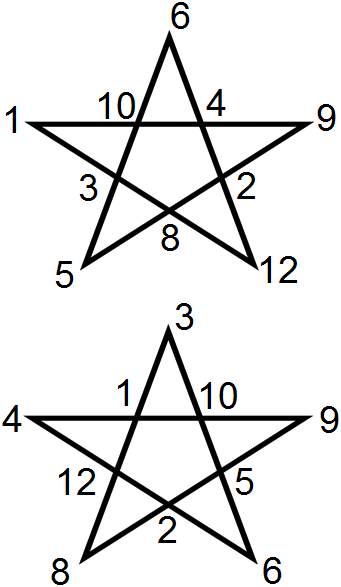BrainDen.com - Brain Teasers
• 0

# star

Go to solution Solved by Logophobic,

## Recommended Posts

• 0
• Solution
Spoiler##### Share on other sites
• 0

9 hours ago, Logophobic said:

but the first answer is wrong, because 1+6+9+5+12 =/= 10+4+2+8+3.

We can easily find the other right answer from the first right answer.
hint : put the inner points to the outer points.

Edited by jasen
##### Share on other sites
• 0

1+6+9+5+12 = 5+12+10+4+2, as required: A+B+C+D+E = D+E+F+G+H

Of course, A+B+C+D+E = F+G+H+I+J does seem more appropriate, but I was working with what you posted.

##### Share on other sites
• 0
13 hours ago, Logophobic said:

1+6+9+5+12 = 5+12+10+4+2, as required: A+B+C+D+E = D+E+F+G+H

Of course, A+B+C+D+E = F+G+H+I+J does seem more appropriate, but I was working with what you posted.

Ok, I just realize my question was wrong, yes it should be A+B+C+D+E = F+G+H+I+J

## Join the conversation

You can post now and register later. If you have an account, sign in now to post with your account.×   Pasted as rich text.   Paste as plain text instead

Only 75 emoji are allowed.

×   Your previous content has been restored.   Clear editor

×   You cannot paste images directly. Upload or insert images from URL.